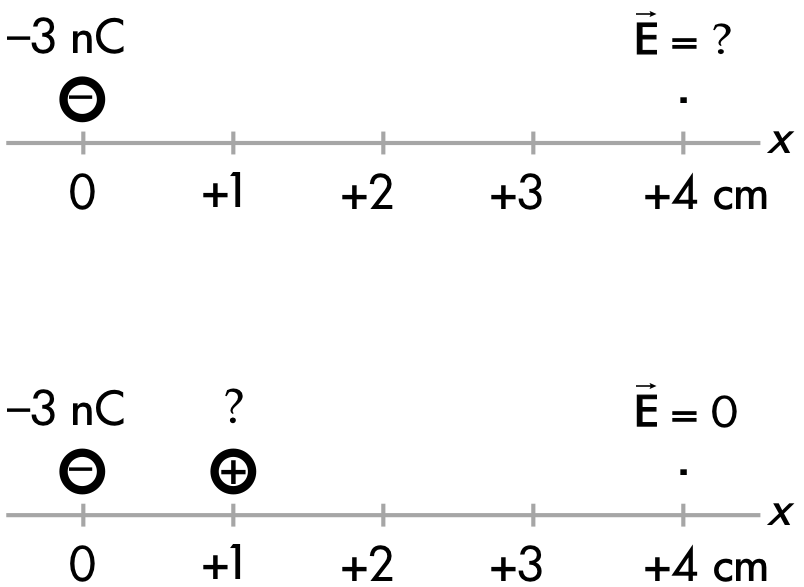## 20180324

### Physics midterm question: charge to "cancel out" electric field

Physics 205B Midterm 1, spring semester 2018
Cuesta College, San Luis Obispo, CAA –3 nC point charge is held at x = 0, such that there is an electric field at x = +4 cm. In order to have no electric field magnitude at x = +4 cm, discuss whether the amount of positive charge to be placed at x = +1 cm should be less than, greater than, or equal to 3 nC. Explain your reasoning using properties of electric forces, fields, and vector superposition.

• p:
Correct. The amount of the (positive) source charge Q2 must be less than 3 nC in order for the (total) electric field magnitude at x = +4 cm to be zero by discussing/demonstrating:
1. that the electric field at x = +4 cm of the Q1 = +3.0 nC source charge pointing to the right (away from this positive source charge) must be "canceled" by the electric field at x = +4 cm of the negative Q2 source charge pointing to the left (in towards this negative source charge), such that the magnitudes of their respective electric fields must be set equal to each other;
2. solves for the (negative) amount of Q2 source charge, which is less than 3.0 nC. (May also place a trial amount of Q2 source charge to demonstrate that it must be less than (−)3.0 nC in order for its electric field to equal the magnitude of the Q1 source charge.)
• r:
As (p), but argument indirectly, weakly, or only by definition supports the statement to be proven, or has minor inconsistencies or loopholes. May not explicitly discuss/demonstrate how the numerical values of the two source charges does not matter.
• t:
Nearly correct, but argument has conceptual errors, or is incomplete.
• v:
Limited relevant discussion of supporting evidence of at least some merit, but in an inconsistent or unclear manner. Some garbled attempt at applying properties of electric forces, fields, and vector superposition.
• x:
Implementation/application of ideas, but credit given for effort rather than merit. No clear attempt at applying properties of electric forces, fields, and vector superposition.
• y:
Irrelevant discussion/effectively blank.
• z:
Blank.
Sections 30882, 30883
Exam code: midterm01cVdP
p: 21 students
r: 5 students
t: 6 students
v: 3 students
x: 0 student
y: 0 students
z: 0 student

A sample "p" response (from student 2525):Another sample "p" response (from student 7744), dropping all common k factors, and common nC and cm units: